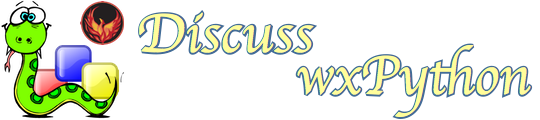# Floating buttons issue

Greetings,

Introduction
I’m working on a kiosk application for a museum that shows an image on a Iiyama 22 inch touchscreen using a raspberry pi and Python/wxPython as programming environment. An Iiyama touch screen is not the same as an iPad or android screen meaning it does not ‘understand’ pinch gestures with two fingers. It only generates left mouse up, left mouse down and mouse move events. Which is fine for most applications.

My Plan
So I decided to make an image viewer with some ordinary buttons for zoom in zoom out, pan to left, right and pan up and down. So far so good because the (incomplete) logic just works. The image is painted on the application frame and the buttons are positioned on a (mostly) transparent panel. So far so good.

The Problem
The problem I need to solve first is that when the zoom-in button is presses the image does zoom in but al buttons but the zoom-in button appear to disappear. Moving the mouse pointer over the ‘hidden’ buttons let them re-appear, so somehow those objects still ‘live’.

Next steps
If the above problem can be solved I will replace the ordinary text buttons with image buttons and refine the zoom-in zoom-out and pan logic. But first things first.

Questions
How can I let the buttons re-appaer after clicking the zoom-in or zoom-out button?
What am I mssing in the setup of this application?
Should I follow a complete different way of working?
Hope to get some suggestions, kind regards, Adriaan.

``````# UNDER CONSTRUCTION
import sys
import wx

DEBUG = False
BTN_WIDTH           = 80
BTN_HEIGHT          = 40
BTN_GAP             = 40
BTN_BOTTOM_MARGIN   = 10
NOVE_DELTA          = 5

"""
Global variables.
"""
monitor_width = 1000                    # default value for debug mode
monitor_height = 700                    # default value for debug mode
image = "groessen.jpg"

class ControlPanel (wx.Panel):
def __init__(self, parent):
super().__init__(parent)
#
needed_width = BTN_WIDTH * 7 + 6 * BTN_GAP
pos_x1 = int((monitor_width - needed_width) / 2)
pos_x2 = int(pos_x1 + BTN_WIDTH + BTN_GAP)
pos_x3 = int(pos_x2 + BTN_WIDTH + BTN_GAP)
pos_x4 = int(pos_x3 + BTN_WIDTH + BTN_GAP)
pos_x5 = int(pos_x4 + BTN_WIDTH + BTN_GAP)
pos_x6 = int(pos_x5 + BTN_WIDTH + BTN_GAP)
pos_x7 = int(pos_x6 + BTN_WIDTH + BTN_GAP)
#
pos_y = monitor_height - (BTN_HEIGHT) - BTN_BOTTOM_MARGIN
if DEBUG:
pos_y = monitor_height - (2 * BTN_HEIGHT) - BTN_BOTTOM_MARGIN
#
zoomin  = wx.Button(self, size=(BTN_WIDTH,BTN_HEIGHT), pos=(pos_x1, pos_y), label="Zoom in")
zoomout = wx.Button(self, size=(BTN_WIDTH,BTN_HEIGHT), pos=(pos_x2, pos_y), label="Zoom out")
up      = wx.Button(self, size=(BTN_WIDTH,BTN_HEIGHT), pos=(pos_x3, pos_y), label="Up")
down    = wx.Button(self, size=(BTN_WIDTH,BTN_HEIGHT), pos=(pos_x4, pos_y), label="Down")
left    = wx.Button(self, size=(BTN_WIDTH,BTN_HEIGHT), pos=(pos_x5, pos_y), label="Left")
right   = wx.Button(self, size=(BTN_WIDTH,BTN_HEIGHT), pos=(pos_x6, pos_y), label="Right")
exit    = wx.Button(self, size=(BTN_WIDTH,BTN_HEIGHT), pos=(pos_x7, pos_y), label="Exit")
#
zoomin.Bind (wx.EVT_BUTTON, self.OnZoomin)
zoomout.Bind (wx.EVT_BUTTON, self.OnZoomout)
up.Bind (wx.EVT_BUTTON, self.OnUp)
down.Bind (wx.EVT_BUTTON, self.OnDown)
left.Bind (wx.EVT_BUTTON, self.OnLeft)
right.Bind (wx.EVT_BUTTON, self.OnRight)
exit.Bind (wx.EVT_BUTTON, self.OnExit)
#
# initial scale
#
self.scale = 1.0

def OnZoomin(self, evt):
if DEBUG:
print("OnZoomin")
#
self.scale += self.scale/100
if DEBUG:
print (self.scale)
self.Parent.OnZoom (self.scale)

def OnZoomout(self, evt):
if DEBUG:
print("OnZoomout")
#
self.scale -= self.scale/100
if self.scale < 1:
self.scale = 1
if DEBUG:
print (self.scale)
self.Parent.OnZoom (self.scale)

def OnUp(self, evt):
if DEBUG:
print("OnUp")
#
if self.scale > 1:
scale_height = self.Parent.ii_height * self.scale
room_to_move_y = int(scale_height - self.Parent.ii_height)
#
# is moveup possible?
#
if abs(self.Parent.top_y) < room_to_move_y:
self.Parent.top_y -= NOVE_DELTA
print ("top_y=" + str(self.Parent.top_y))
self.Parent.OnZoom (self.scale)

def OnDown(self, evt):
if DEBUG:
print("OnDown")
#
if self.scale > 1:
#
# is movedown possible?
#
if self.Parent.top_y < 0:
self.Parent.top_y += NOVE_DELTA
print ("top_y=" + str(self.Parent.top_y))
self.Parent.OnZoom (self.scale)

def OnLeft(self, evt):
if DEBUG:
print("OnLeft")
#
# is move left possible?
#
if self.scale > 1:
scale_width = self.Parent.ii_width * self.scale
room_to_move_x = int(scale_width - self.Parent.ii_width )
if abs(self.Parent.top_x) < room_to_move_x:
self.Parent.top_x -= NOVE_DELTA
print ("top_x=" + str(self.Parent.top_x))
self.Parent.OnZoom (self.scale)

def OnRight(self, evt):
if DEBUG:
print("OnRight")
#
# is move right possible?
#
if self.Parent.top_x < 0:
self.Parent.top_x += NOVE_DELTA
print ("top_x=" + str(self.Parent.top_x))
self.Parent.OnZoom (self.scale)

def OnExit(self, evt):
if DEBUG:
print("OnExit")
#
sys.exit()

class MainFrame(wx.Frame):
def __init__(self, *args, **kw):
super(MainFrame, self).__init__(*args, **kw)
#
img = wx.Image(image).Scale(monitor_width, monitor_height)
self.bm = wx.Bitmap(img, wx.BITMAP_SCREEN_DEPTH)
self.Bind (wx.EVT_PAINT, self.OnPaint)
#
self.ii_height = img.Height
self.ii_width  = img.Width
self.top_x = 0
self.top_y = 0
#
cp = ControlPanel(self)
cp.SetWindowStyle(wx.TRANSPARENT_WINDOW)
#
if DEBUG:
self.SetSize(monitor_width, monitor_height)
self.SetPosition((0,0))
self.Show()
else:
self.ShowFullScreen(True)

def OnPaint(self, evt):
if DEBUG:
print("OnPaint")
#
dc = wx.PaintDC(self)
dc.DrawBitmap(self.bm, 0, 0)

def OnZoom (self, scale):
if DEBUG:
print ("OnZoom")
#
dc = wx.WindowDC(self)
dc.SetUserScale(scale, scale)
dc.DrawBitmap(self.bm, self.top_x, self.top_y)

if __name__ == '__main__':
app = wx.App()
if DEBUG:
pass                            # leave the screen size as defined in global vars
else:
monitor = wx.GetDisplaySize()   # get the full size of this monitor
monitor_width = monitor
monitor_height = monitor
#
mf = MainFrame(None)
app.MainLoop()

``````

When you re-draw your image, you paint over your buttons. If you call Refresh() on each button after re-drawing your image, the buttons will re-draw themselves too. Here’s the code I changed:

``````class MainFrame(wx.Frame):
def __init__(self, *args, **kw):
super(MainFrame, self).__init__(*args, **kw)``````

Thank you DavidW,

But those two lines of code are identical. Maybe copy paste glitch?

Thank you DavidW,

Got it working with .Refresh() !

Definitely an e-mail glitch. The version in my “Sent Mail” folder contains more code than came through to the list.

The critical lines, at the end of your OnZoom() method after changing cp to self.cp in init(), are:

``````       <b>children = self.cp.GetChildren()
for child in children:
child.Refresh()

</b>
``````

Sorry about that. I hope it comes through this time.

David

1 Like